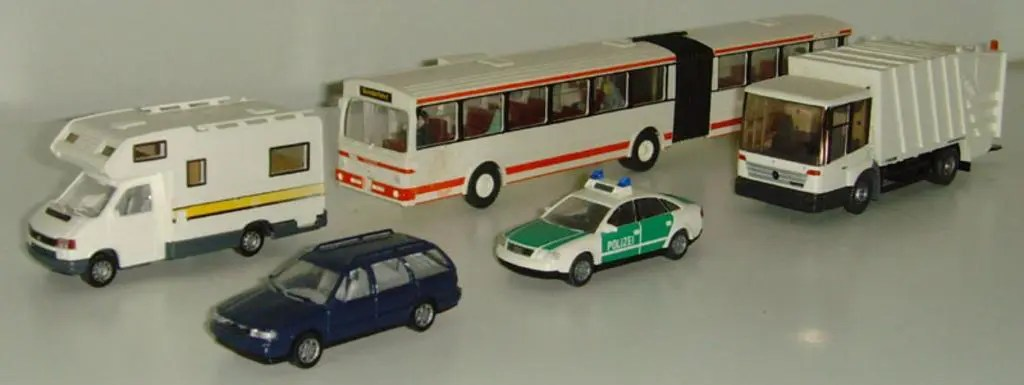# Which is bigger, 1:64 scale or 1:87 scale

Scale can be a confusing concept to explain or to understand. So it’s very common to hear questions like which is bigger, 1:64 scale or 1:87 scale. I’ll try to explain it simply.

Scale is a ratio between the size of a model or toy and the real thing it represents. A 1:64 scale model is 1/64 the size of the real thing. A 1:87 model is 1/87 the size of the real thing. In scale, higher numbers are smaller.

## How are big numbers smaller?When models are all the same scale, relative size remains in proportion so a truck is larger than a passenger car.

Remember, scale is a ratio. If it takes 64 models to equal the size of the real thing, that means the models are bigger than one that takes 87 to equal the size of the real thing. I find it helps to remember it that way.

Keeping models in the same scale keeps sizes in proportion. A 1:64 scale passenger car truck-sized in comparison with a 1:87 model of the same car.

## How scale is calculated

To calculate scale, you measure the real thing, then reduce it. A common example is to divide the size by 10 or 100. If you use metric measurements, this is very easy and convenient to calculate.

The reason many scales aren’t even multiples of 10 come from calculating scale using imperial measurements. When you make an inch represent one foot in the real thing, you end up with 1:12 scale. Using fractions of an inch results in scales like 1:64 or 1:48. Using smaller metric units to represent imperial units results in scale sizes like 1:43 or 1:87.